# Miscellaneous Solved Problems in Modular Programming - LabVIEW

Rating: 4

4230

1. Create a VI to compute full adder logic using half adder logic as subVI.
Solution:  The front panel and the block diagrams for the half adder is shown in Figures P3.1(a) and P3.1(b). Create a subVI called “HALF” and use this in the main VI to create a full adder as given in Figures P3.1(c) and P3.1(d).

If you would like to Enrich your career with an Android certified professional, then visit Mindmajix - A Global online training platform: “LabVIEW training” Course.This course will help you to achieve excellence in this domain.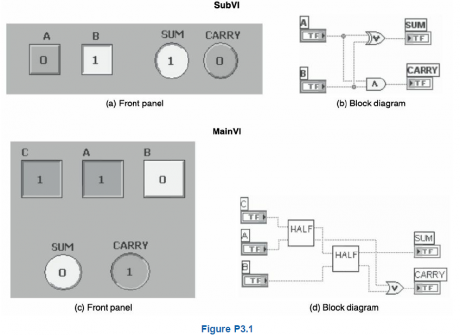2.Create a VI to find the decimal equivalent of a binary number using subVI.
Solution: To find the decimal equivalent of a binary number, first create the front panel and the block diagram as given in Figures P3.2(a) and P3.2(b). Then create the subVI called “Binary to decimal” and use it in the main VI as shown in Figures P3.2(c) and P3.2(d).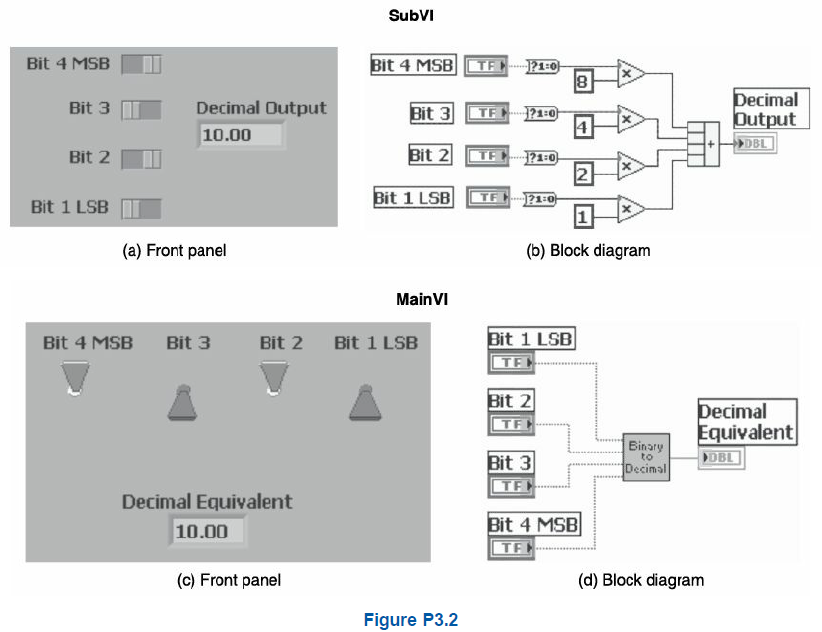3.Create a VI to find the Grey code equivalent of a BCD number using subVIs.
Solution: Create the front panel and the block diagram as given in Figure P3.3(a) and P3.3(b). Then create the subVI called “BCD to GRAY” and use it in the main VI as shown in Figure P3.3(c) and P3.3(d).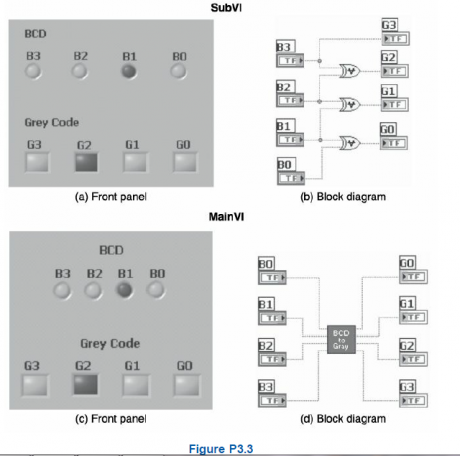4.Create a VI to find the roots of a quadratic equation using subVIs. Find both the values of the roots and the nature of the roots.
Solution: Create the front panel and the block diagram as shown in Figures P3.4(a) and P3.4(b). Then create the subVI and use it in the main VI as shown in Figures P3.4(c) and P3.4(d).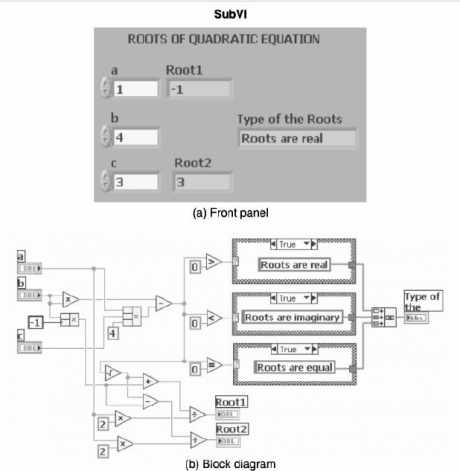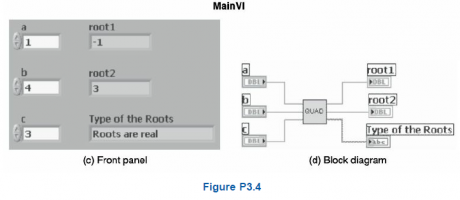5.Create a VI to find the average of two numbers and convert a section of a VI into a subVI.
Solution: The block diagram to find the average of two numbers and convert a section of a VI into a subVI by selecting that portion is shown in Figures P3.5(a) and P3.5(b) respectively.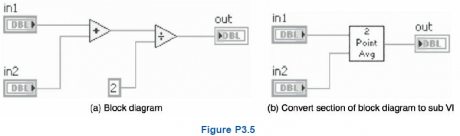6.Create the front panel and block diagram of the Main VI to show the trigonometric values (sine, cosine and tangent) of the given degree. This VI uses a subVI for finding the trigonometric values. The SubVI consists of functions for converting degree values to radians and functions to find the sine, cosine and tangent values separately. In LabVIEW the sine, cosine and tangent functions take input in radians.
Solution:  Create the front panel and the block diagram as shown in Figures (a) and (b).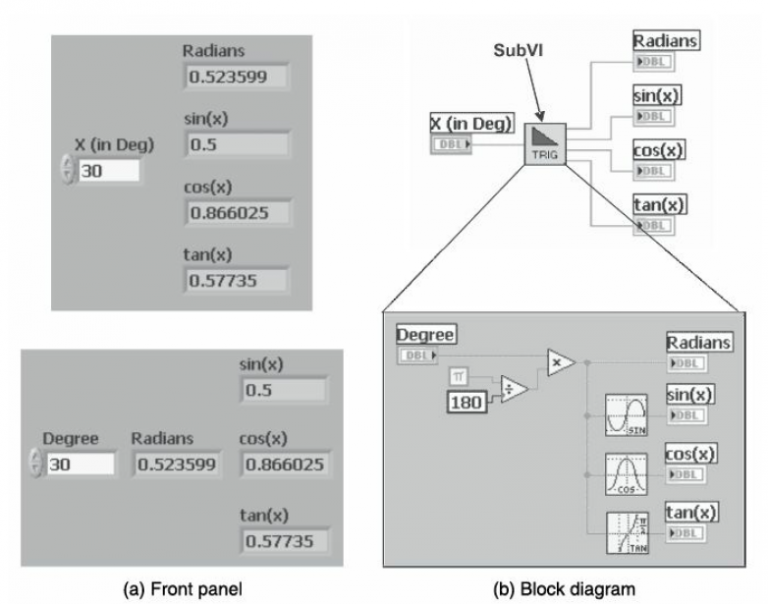Check Out LabVIEW TutorialsCourse Schedule
NameDates
LabVIEW TrainingFeb 11 to Feb 26
LabVIEW TrainingFeb 14 to Mar 01
LabVIEW TrainingFeb 18 to Mar 05
LabVIEW TrainingFeb 21 to Mar 08
Last updated: 09 February 2023